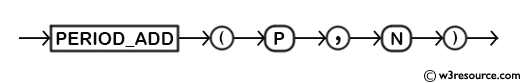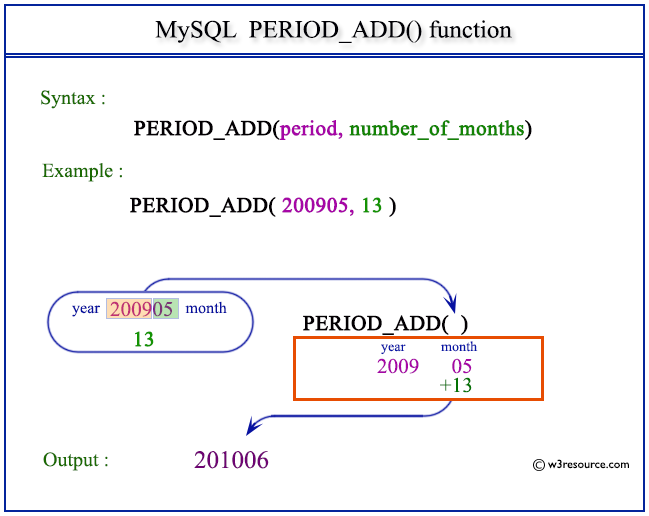MySQL PERIOD_ADD() adds a number of months with a period and returns the value in the format YYYYMM OR YYMM. Remember that the format YYYYMM and YYMM are not date values. It provides a way to perform period arithmetic, which is particularly useful when dealing with data that is represented in periods (year-month intervals).

This function is useful in -

• The function is used to project periods into the future or past based on specified increments.
• PERIOD_ADD() aids in financial analysis that involves projecting future periods for planning and forecasting.
• The function is valuable for analyzing trends and patterns over extended periods by projecting time intervals.
• PERIOD_ADD() is used to aggregate data over projected periods, helping to create time-based summaries.
• PERIOD_ADD() can be used in scenarios where period values need to be transformed for specific purposes.
• PERIOD_ADD() is valuable for calculating future or past periods by adding a specific number of months to an existing period.

Syntax:

```PERIOD_ADD(P,N);
```

Arguments:

Name Description
P Indicates a period.
N Indicates a number of months.

Syntax Diagram:MySQL Version: 8.0

Pictorial Presentation:The following statement will return a value in YYYYMM or YYMM format after adding 13 months with the period 200905.

Code:

``````SELECT PERIOD_ADD(200905,13);
```
```

Output:

```mysql> SELECT PERIOD_ADD(200905,13);
+-----------------------+
+-----------------------+
|                201006 |
+-----------------------+
1 row in set (0.00 sec)
```

Video Presentation:

All Date and Time Functions: